# Integral of 1/sqrt(e^x-1) from ln(2) to infinityGraph of the function with the red shaded region being the area we are going to calculate.

Hi guys! Hope you are all doing well in these days of quarantine which is hopefully ending soon. I like to spend my time integrating and watching videos about calculus in general on Youtube. Blackpenredpen is one of my favourite channels, and this is where I take some of my ideas. What I do is I see what the problem is, pause the video and try to solve it. Then I watch it to see whether I used the same procedure and if the result is correct. Recently I came up with this integral (link to the video here):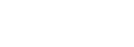Try to solve it first and let me know in the comments if you got it right!

Ok, so, don’t be intimidated by the bounds. Let’s first treat it as an indefinite integral. We are going to use u-substitution. Usually, when there is a square root, it’s better to let u be the whole square root. You’ll see why: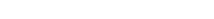As you can see the square root is gone, since we squared both sides. Now we can take the derivative of each side and find dx: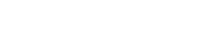Now, since we are performing a u-substitution, we want everything to be in terms of u. Usually something like this happens: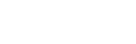The extra term in x simplifies, but this is not the case in our integral, therefore the only way to bring everything to the u-world is to write e^x in terms of u: we know that u²=e^x-1, hence e^x=u²+1.

Overall,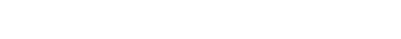Before we substitute though, we must change the bounds, since those are the values of x: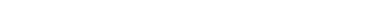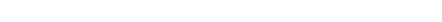We are now ready to substitute: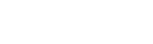As you can see, the u‘s cancel out; also, the 2 is brought to the front of the integral (linearity):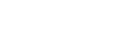This is an integral you should remember: the result is the inverse tangent: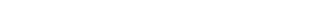You should either remember the angles or search them up: the tangent of pi/2 (90°) is infinity and that of pi/4 (45°) is 1, therefore the inverse tangents of infinity and 1 are respectively pi/2 and pi/4: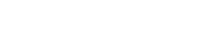pi/2 is the result. Isn’t that cool?

If you are interested in an explanation about u-substitution, check these out:

u-substitution part 1

u-substitution part 2

I really hope everything was clear, and if you have any doubts, questions or suggestions leave a comment and I’ll be happy to reply! If you liked this post give it a like and subscribe to stay updated!

Join 31 other subscribers.